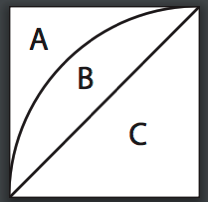Posted on

# Geometry Ch14 Test #4

Question: how do we work the problem on Chapter 14 test #4.

Since all 16 white regions are identical, we can find the area of one of them and multiply it by 16.

This figure represents one of the 16 small squares and one of the white regions.Since the diameter of the large circle in the figure shown on the test page is 4 units, the side of the square of one of the small squares is 1 unit.

We can find the area of region B by finding the area of regions B & C combined (a quarter of a circle) and subtracting the area of region C (a triangle):

> The area of B & C combined is1/4 (pi r squared) or 1/4 (pi 1 squared) = 1/4 pi

> The area of C is1/2 r squared or 1/2 1 squared = 1/2So B = (B + C) – C = 1/4 pi – 1/2 and the total area of the 16 white regions is16(1/4 pi – 1/2) = 4 pi – 8.